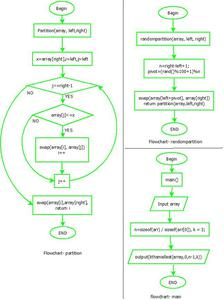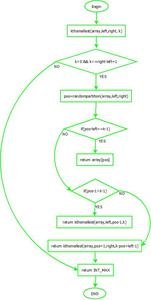Open in App
Not now

# K’th Smallest/Largest Element in Unsorted Array | Set 2 (Expected Linear Time)

• Difficulty Level : Medium
• Last Updated : 14 Dec, 2022

Given an array and a number k where k is smaller than the size of the array, we need to find the k’th largest element in the given array. It is given that all array elements are distinct.

We recommend reading the following post as a prerequisite to this post. K’th Smallest/Largest Element in Unsorted Array | Set 1

Examples:

```Input: arr[] = {7, 10, 4, 3, 20, 15}
k = 3
Output: 7

Input: arr[] = {7, 10, 4, 3, 20, 15}
k = 4
Output: 10```

We have discussed three different solutions here. In this post method 4, it is discussed which is mainly an extension of method 3 (QuickSelect) discussed in the previous post.

The flowchart is as follows:Flowchartflowchart- kth smallest

## C++

 `// C++ program of above implementation` `#include ``using` `namespace` `std;` `// Standard partition process of QuickSort().``// It considers the last element as pivot and``// oves all smaller element to left of it``// and greater elements to right``int` `partition(``int``* arr, ``int` `l, ``int` `r)``{``    ``int` `x = arr[r], i = l;``    ``for` `(``int` `j = l; j <= r - 1; j++) {``        ``if` `(arr[j] <= x) {``            ``swap(arr[i], arr[j]);``            ``i++;``        ``}``    ``}``    ``swap(arr[i], arr[r]);``    ``return` `i;``}` `int` `randomPartition(``int``* arr, ``int` `l, ``int` `r)``{``    ``int` `n = r - l + 1;``    ``int` `pivot = (``rand``() % 100 + 1) % n;``    ``swap(arr[l + pivot], arr[r]);``    ``return` `partition(arr, l, r);``}` `// This function returns k'th smallest``// element in arr[l..r] using``// QuickSort based method. ASSUMPTION:``// ALL ELEMENTS IN ARR[] ARE DISTINCT``int` `kthSmallest(``int``* arr, ``int` `l, ``int` `r, ``int` `k)``{` `    ``// If k is smaller than number``    ``// of elements in array``    ``if` `(k > 0 && k <= r - l + 1) {` `        ``// Partition the array around last``        ``// element and get position of pivot``        ``// element in sorted array``        ``int` `pos = randomPartition(arr, l, r);` `        ``// If position is same as k``        ``if` `(pos - l == k - 1) {``            ``return` `arr[pos];``        ``}` `        ``// If position is more, recur``        ``// for left subarray``        ``if` `(pos - l > k - 1) {``            ``return` `kthSmallest(arr, l, pos - 1, k);``        ``}` `        ``// Else recur for right subarray``        ``return` `kthSmallest(arr, pos + 1, r,``                           ``k - pos + l - 1);``    ``}` `    ``// If k is more than number of``    ``// elements in array``    ``return` `INT_MAX;``}` `// Driver Code``int` `main()``{``    ``int` `arr[] = { 12, 3, 5, 7, 4, 19, 26 };``    ``int` `n = ``sizeof``(arr) / ``sizeof``(arr), k = 3;``    ``cout << ``"K'th smallest element is "``         ``<< kthSmallest(arr, 0, n - 1, k);``}`

## Java

 `// Java program of above implementation` `import` `java.util.Random;` `public` `class` `GFG {` `// This function returns k'th smallest element in arr[l..r] using``// QuickSort based method. ASSUMPTION: ALL ELEMENTS IN ARR[] ARE DISTINCT``    ``static` `int` `kthSmallest(``int` `arr[], ``int` `l, ``int` `r, ``int` `k) {``        ``// If k is smaller than number of elements in array``        ``if` `(k > ``0` `&& k <= r - l + ``1``) {``            ``// Partition the array around last element and get``            ``// position of pivot element in sorted array``            ``int` `pos = randomPartition(arr, l, r);` `            ``// If position is same as k``            ``if` `(pos - l == k - ``1``) {``                ``return` `arr[pos];``            ``}``            ``if` `(pos - l > k - ``1``) ``// If position is more, recur for left subarray``            ``{``                ``return` `kthSmallest(arr, l, pos - ``1``, k);``            ``}` `            ``// Else recur for right subarray``            ``return` `kthSmallest(arr, pos + ``1``, r, k - pos + l - ``1``);``        ``}` `        ``// If k is more than number of elements in array``        ``return` `Integer.MAX_VALUE;``    ``}` `    ``static` `void` `swap(``int``[] arr, ``int` `i, ``int` `j) {``        ``int` `temp = arr[i];``        ``arr[i] = arr[j];``        ``arr[j] = temp;``    ``}` `// Standard partition process of QuickSort(). It considers the last``// element as pivot and moves all smaller element to left of it``// and greater elements to right``    ``static` `int` `partition(``int` `arr[], ``int` `l, ``int` `r) {``        ``int` `x = arr[r], i = l;``        ``for` `(``int` `j = l; j <= r - ``1``; j++) {``            ``if` `(arr[j] <= x) {``                ``swap(arr, i, j);``                ``i++;``            ``}``        ``}``        ``swap(arr, i, r);``        ``return` `i;``    ``}` `    ``static` `int` `randomPartition(``int` `arr[], ``int` `l, ``int` `r) {``        ``int` `n = r - l + ``1``;``        ``int` `pivot = ``new` `Random().nextInt(n);``        ``swap(arr, l + pivot, r);``        ``return` `partition(arr, l, r);``    ``}` `// Driver program to test above methods``    ``public` `static` `void` `main(String args[]) {``        ``int` `arr[] = {``12``, ``3``, ``5``, ``7``, ``4``, ``19``, ``26``};``        ``int` `n = arr.length, k = ``3``;``        ``System.out.println(``"K'th smallest element is "` `+ kthSmallest(arr, ``0``, n - ``1``, k));``    ``}``}` `/*This code is contributed by 29AjayKumar*/`

## Python3

 `# Python3 implementation of above implementation` `# This function returns k'th smallest element``# in arr[l..r] using QuickSort based method.``# ASSUMPTION: ALL ELEMENTS IN ARR[] ARE DISTINCT``from` `random ``import` `randint` `def` `randomPartition(arr, l, r):``    ``n ``=` `r ``-` `l ``+` `1``    ``pivot ``=` `randint(``1``, ``100``) ``%` `n``    ``arr[l ``+` `pivot], arr[r] ``=` `arr[l ``+` `pivot], arr[r]``    ``return` `partition(arr, l, r)` `def` `kthSmallest(arr, l, r, k):` `    ``# If k is smaller than``    ``# number of elements in array``    ``if` `(k > ``0` `and` `k <``=` `r ``-` `l ``+` `1``):` `        ``# Partition the array around last element and``        ``# get position of pivot element in sorted array``        ``pos ``=` `randomPartition(arr, l, r)` `        ``# If position is same as k``        ``if` `(pos ``-` `l ``=``=` `k ``-` `1``):``            ``return` `arr[pos]``            ` `        ``# If position is more, recur for left subarray``        ``if` `(pos ``-` `l > k ``-` `1``):``            ``return` `kthSmallest(arr, l, pos ``-` `1``, k)` `        ``# Else recur for right subarray``        ``return` `kthSmallest(arr, pos ``+` `1``, r,``                           ``k ``-` `pos ``+` `l ``-` `1``)` `    ``# If k is more than number of elements in array``    ``return` `10``*``*``9` `# Standard partition process of QuickSort().``# It considers the last element as pivot and``# moves all smaller element to left of it``# and greater elements to right``def` `partition(arr, l, r):``    ``x ``=` `arr[r]``    ``i ``=` `l``    ``for` `j ``in` `range``(l, r):``        ``if` `(arr[j] <``=` `x):``            ``arr[i], arr[j] ``=` `arr[j], arr[i]``            ``i ``+``=` `1` `    ``arr[i], arr[r] ``=` `arr[r], arr[i]``    ``return` `i` `# Driver Code``arr ``=` `[``12``, ``3``, ``5``, ``7``, ``4``, ``19``, ``26``]``n ``=` `len``(arr)``k ``=` `3``print``(``"K'th smallest element is"``,``       ``kthSmallest(arr, ``0``, n ``-` `1``, k))` `# This code is contributed by Mohit Kumar`

## C#

 `// C# program of above implementation` `using` `System;` `class` `GFG``{` `// This function returns k'th smallest``// element in arr[l..r] using``// QuickSort based method. ASSUMPTION:``// ALL ELEMENTS IN ARR[] ARE DISTINCT``static` `int` `kthSmallest(``int` `[]arr, ``int` `l,``                       ``int` `r, ``int` `k)``{``    ``// If k is smaller than number``    ``// of elements in array``    ``if` `(k > 0 && k <= r - l + 1)``    ``{``        ``// Partition the array around last``        ``// element and get position of pivot``        ``// element in sorted array``        ``int` `pos = randomPartition(arr, l, r);` `        ``// If position is same as k``        ``if` `(pos - l == k - 1)``        ``{``            ``return` `arr[pos];``        ``}``        ` `        ``// If position is more, recur``        ``// for left subarray``        ``if` `(pos - l > k - 1)``        ``{``            ``return` `kthSmallest(arr, l, pos - 1, k);``        ``}` `        ``// Else recur for right subarray``        ``return` `kthSmallest(arr, pos + 1, r,``                           ``k - pos + l - 1);``    ``}` `    ``// If k is more than number of``    ``// elements in array``    ``return` `int``.MaxValue;``}` `static` `void` `swap(``int``[] arr, ``int` `i, ``int` `j)``{``    ``int` `temp = arr[i];``    ``arr[i] = arr[j];``    ``arr[j] = temp;``}` `// Standard partition process of QuickSort().``// It considers the last element as pivot and``// oves all smaller element to left of it``// and greater elements to right``static` `int` `partition(``int` `[]arr, ``int` `l, ``int` `r)``{``    ``int` `x = arr[r], i = l;``    ``for` `(``int` `j = l; j <= r - 1; j++)``    ``{``        ``if` `(arr[j] <= x)``        ``{``            ``swap(arr, i, j);``            ``i++;``        ``}``    ``}``    ``swap(arr, i, r);``    ``return` `i;``}` `static` `int` `randomPartition(``int` `[]arr, ``int` `l, ``int` `r)``{``    ``int` `n = r - l + 1;``    ``int` `pivot = ``new` `Random().Next(1);``    ``swap(arr, l + pivot, r);``    ``return` `partition(arr, l, r);``}` `// Driver Code``public` `static` `void` `Main()``{``    ``int` `[]arr = {12, 3, 5, 7, 4, 19, 26};``    ``int` `n = arr.Length, k = 3;``    ``Console.WriteLine(``"K'th smallest element is "` `+``                    ``kthSmallest(arr, 0, n - 1, k));``}``}` `// this code is contributed by 29AjayKumar`

## Javascript

 ``

Output

`K'th smallest element is 5`

Time Complexity : O(N * log N).
Auxiliary Space: O(1).